# Electronic Tic-Tac-Toe

5 May 2007
Progress: Completed

My GCSE Systems & Control project. Far more complicated than needed, but hey, I had a lot of spare time back then.

The original write-up is in Welsh, and after years of thinking "I'll translate it later" I've decided to just post the pictures with minimal explanation.

The LEDs go red for a cross, and green for a naught.

9 input switches, 9 tricolour LEDs as output. The biggest chip I was allowed to use was the PICAXE-18X, with 9 output pins. The tricolour LEDs obviously needed two pins each, so after experimenting with linking multiple chips together and things like that, I stumbled upon an article explaining how to matrix LEDs – which solved the problem perfectly.

Essentially you place the LEDs in a grid, and illuminate one row/column at a time, and cycle through the matrix so fast that it appears they're all lit, thanks to persistence-of-vision. My grid was 3x6, the 6 being the reds and greens, the 3 being the ground wires.

My solution for the inputs was to place a different resistor on each and connect them all to the same analogue input pin. This would then work out which had been pressed from the reading. In retrospect, it would have been easier to just matrix the switches too.

Sadly the analogue readings varied greatly depending on battery life, so I had to add a calibration step when you turned the thing on. Less than ideal, but functional.

I was pretty pleased with this when I finished, but there are infinitely many ways it could have been better. Anyway, pictures.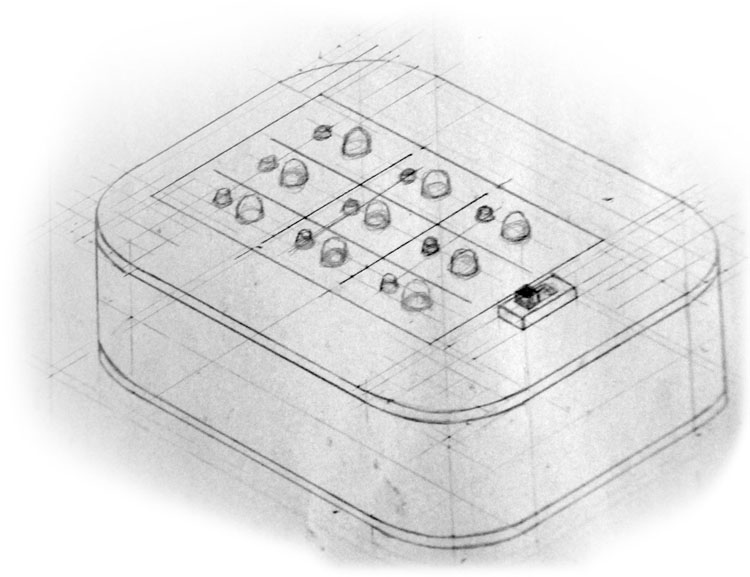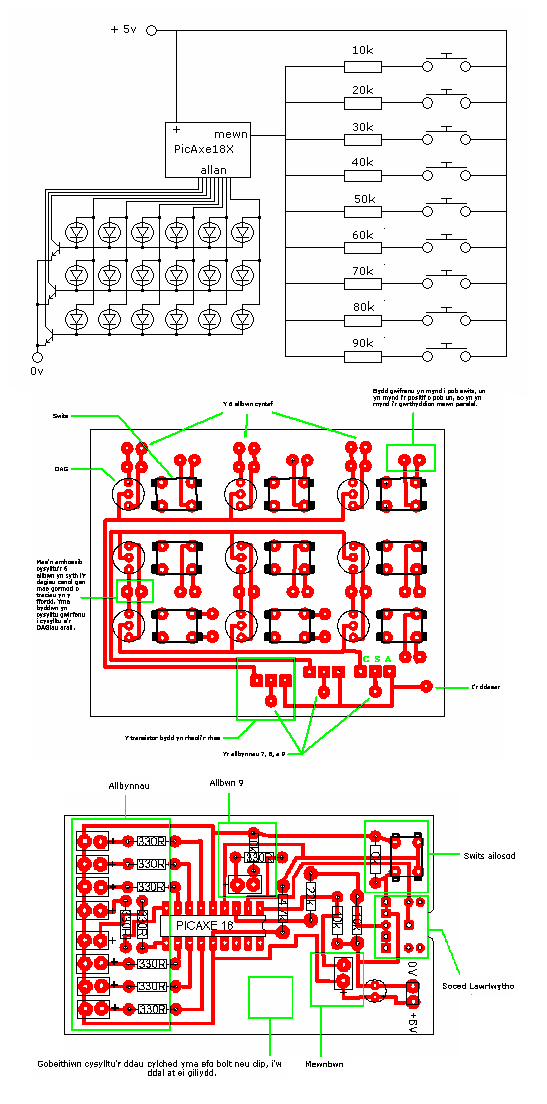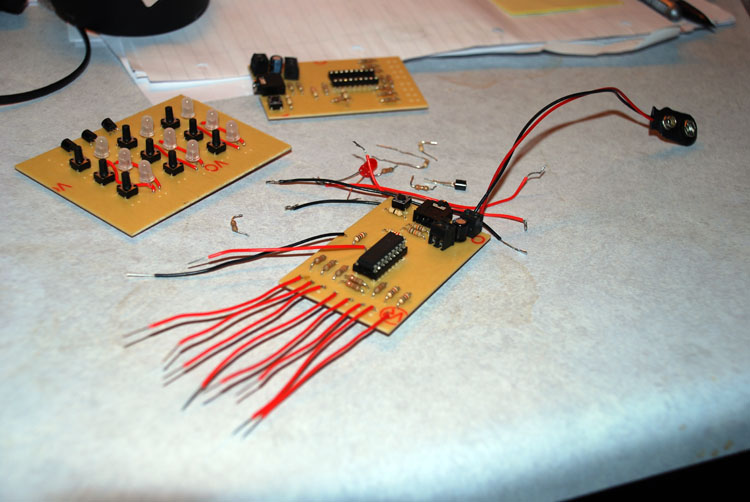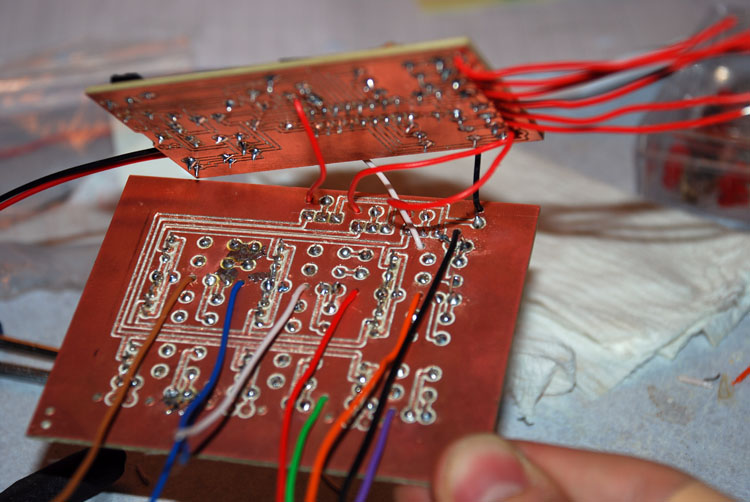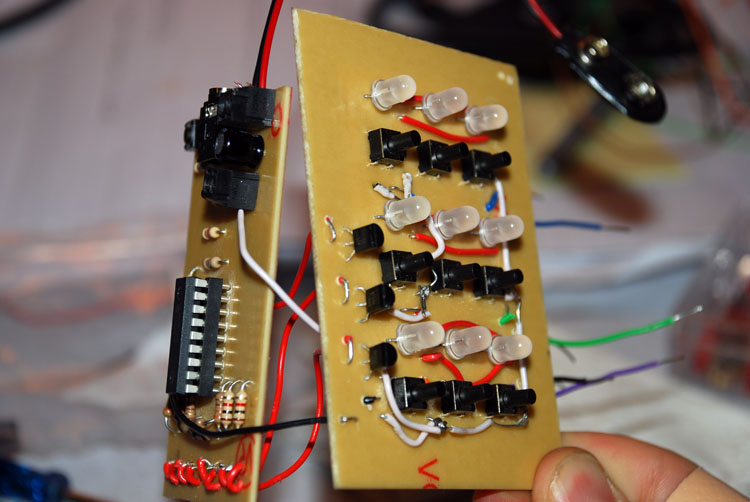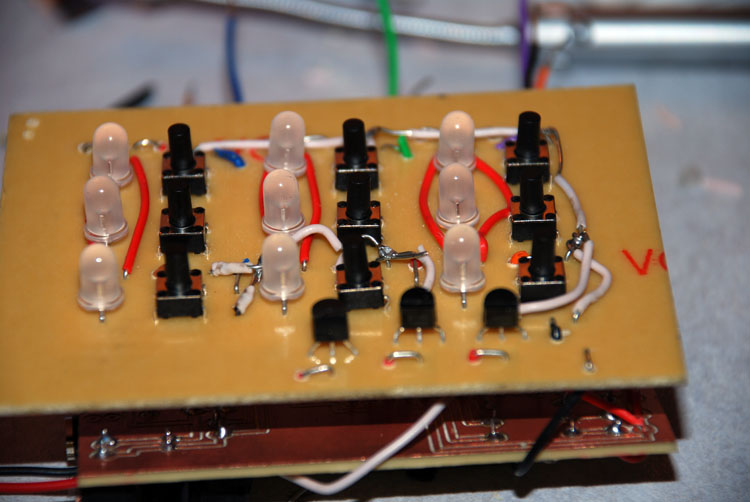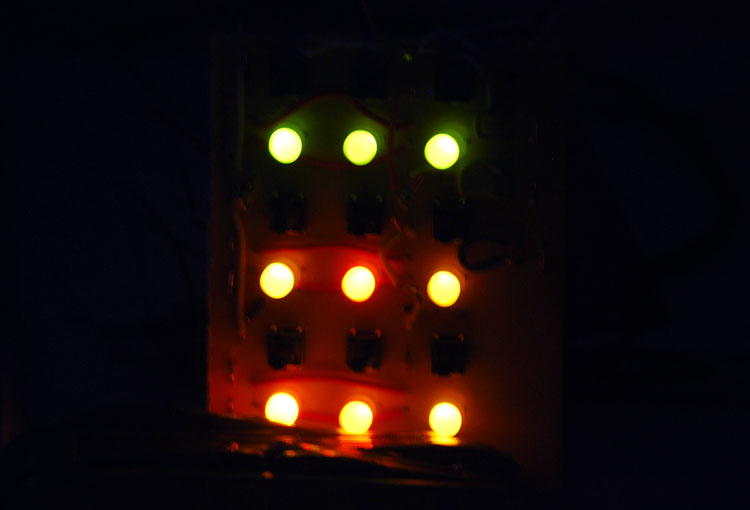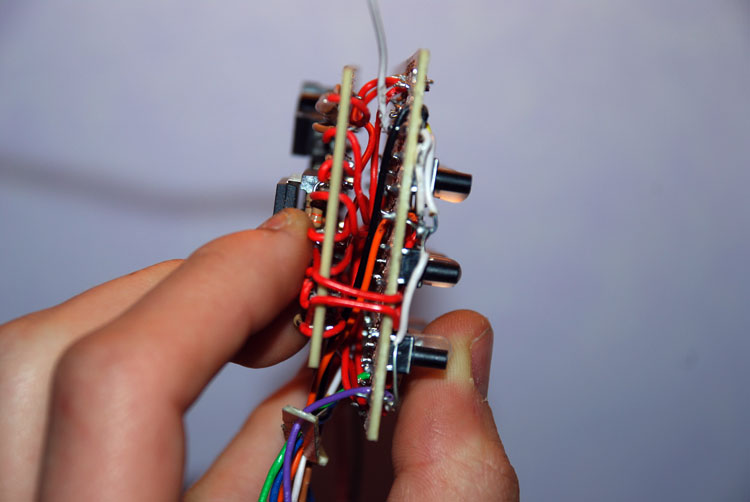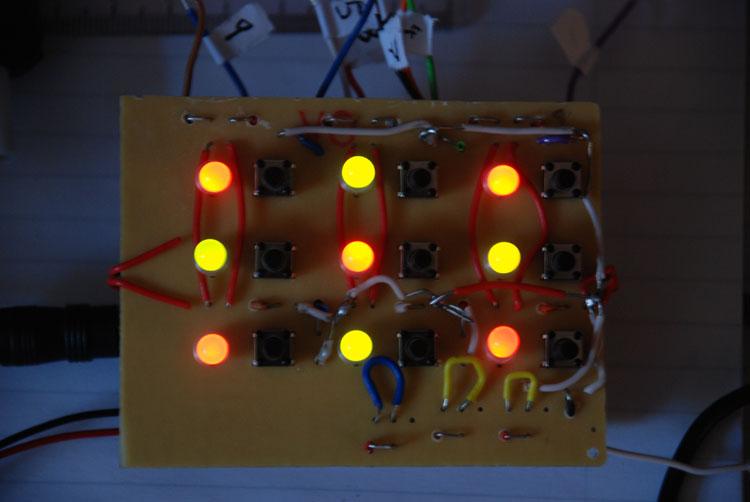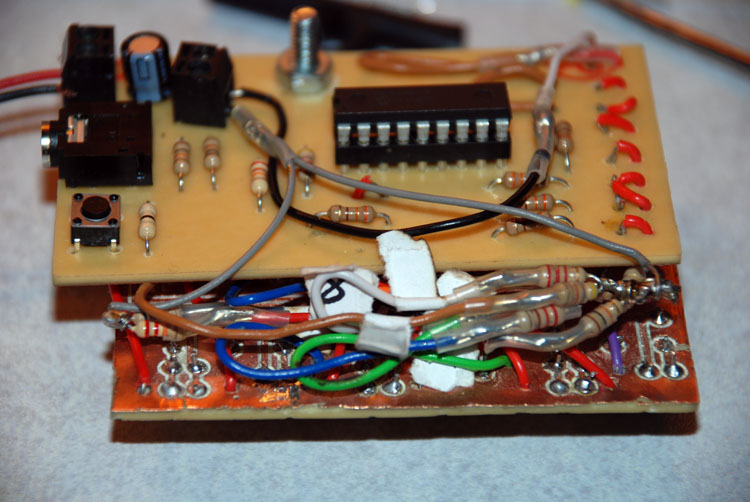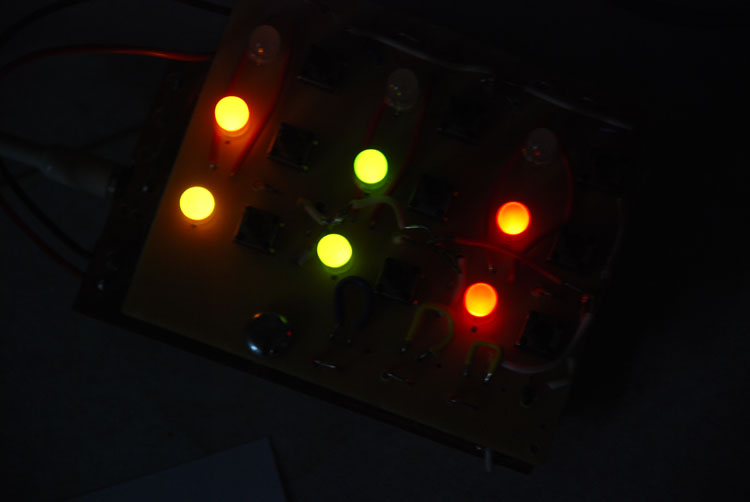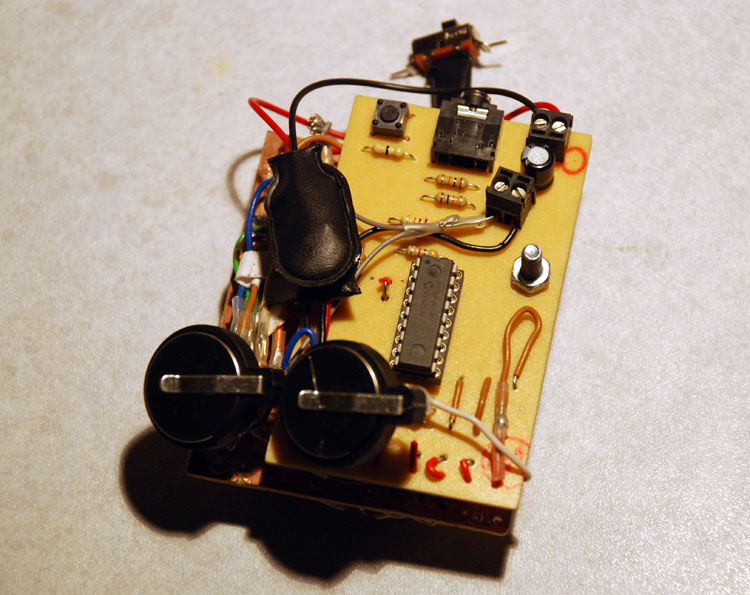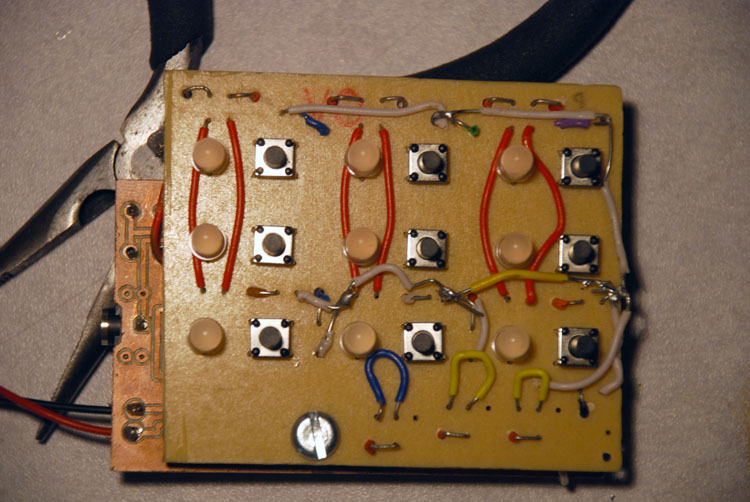This doesn't really do justice to the amount of effort it took to get the matrix working.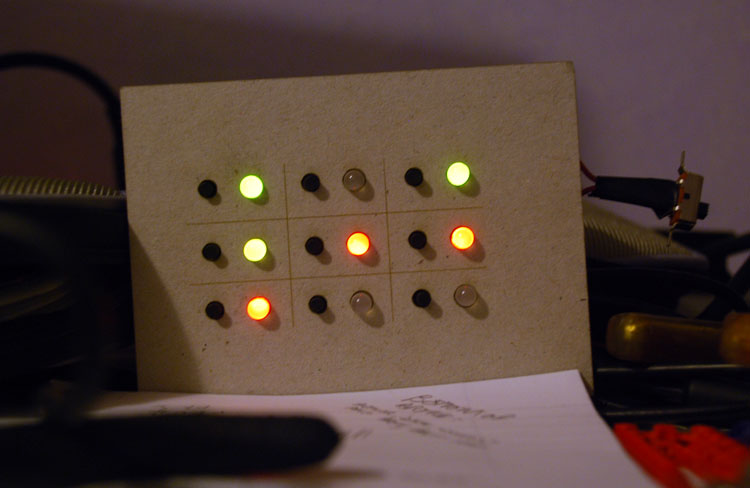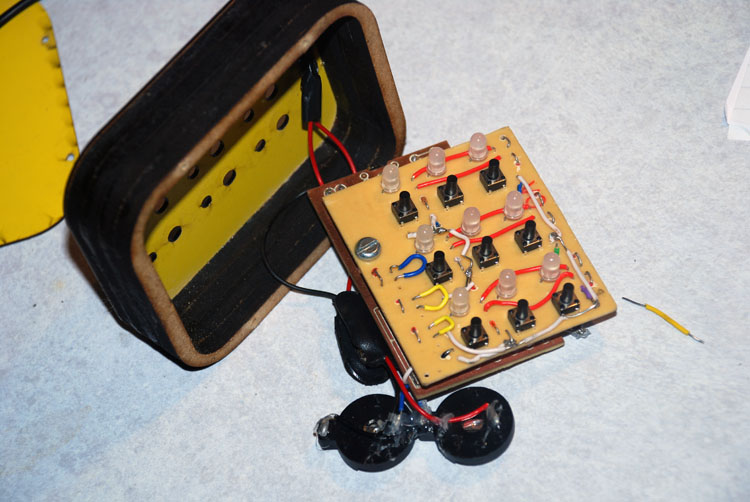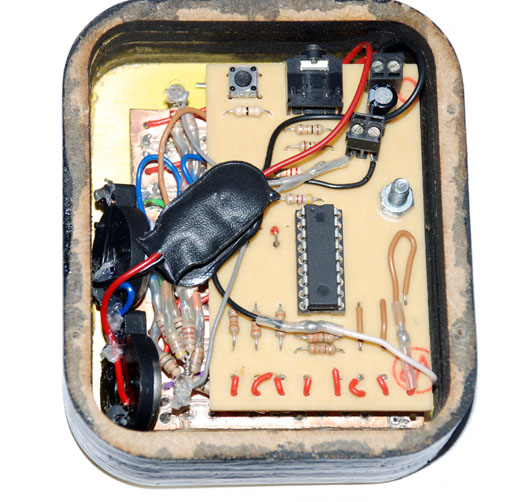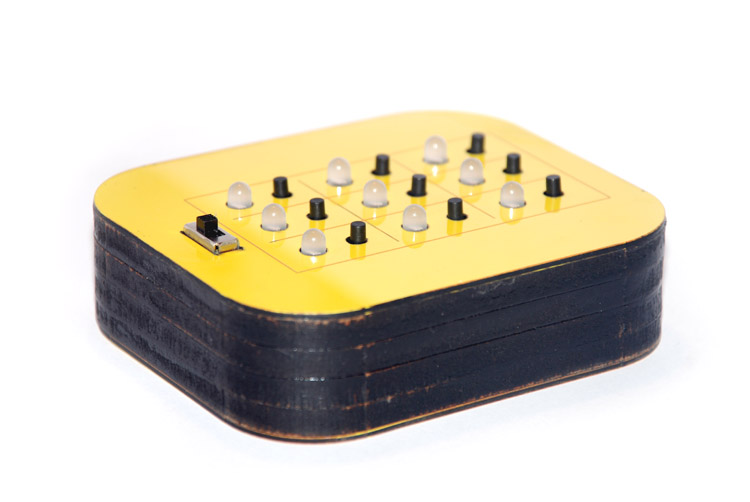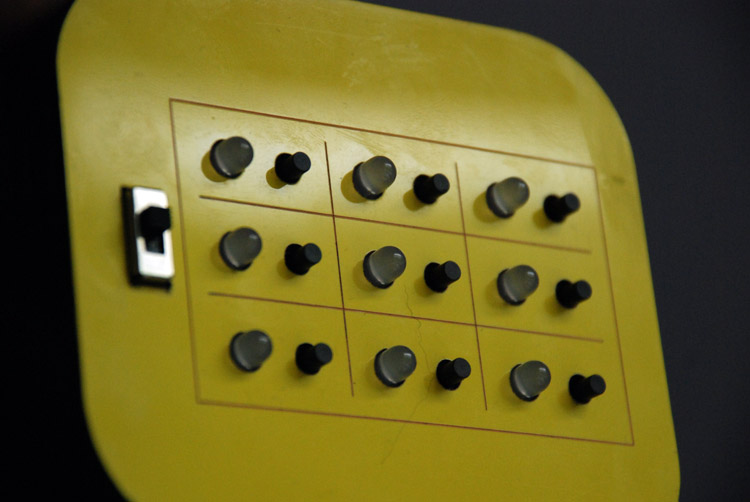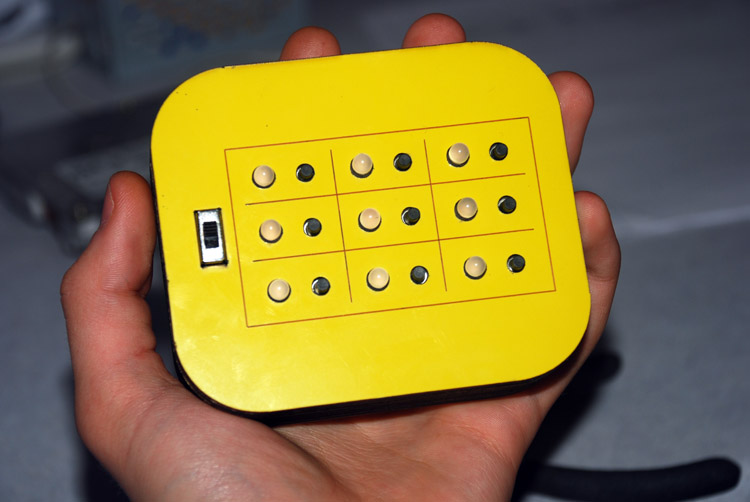I had never used a picaxe before, but the Basic they're programmed with is pretty simple. The final code only just fit on the chip, with something like 5 bytes of free space.
```

''' Roar control of outputs.
' %
' 0 mid row negative
' 0 bottom row negative
' 0 top row negative
' 0  G3
' 0  R2
' 0  G2
' 0  R1
' 0  G1

' SERTXD R3

' % 0   0   0   0   0   0   0   0
'   128 64  32  16  8   4   2   1

''' Finalized control of outputs
' b10 = top row
' b11 = mid row
' b12 = bot row
' %
' 0  - Leave blank
' 0  - Leave blank
' 0  R3
' 0  G3
' 0  R2
' 0  G2
' 0  R1
' 0  G1

main:

symbol TopRow = b10
symbol MidRow = b11
symbol BotRow = b12

symbol inp1 = b1
let inp1 = 0
symbol inp2 = b2
let inp2 = 0
symbol inp3 = b3
let inp3 = 0
symbol inp4 = b4
let inp4 = 0
symbol inp5 = b5
let inp5 = 0
symbol inp6 = b6
let inp6 = 0
symbol inp7 = b7
let inp7 = 0
symbol inp8 = b8
let inp8 = 0
symbol inp9 = b9
let inp9 = 0

'Calibrate inputs
calib1:
let pins = %10100011
poke \$05,%00000000
if b0 = 0 then goto calib1
do while b0 <> 0
if b0>inp1 then
let inp1 = b0
endif
loop

calib2:
let pins = %10101100
poke \$05,%00000000
if b0 = 0 then goto calib2
do while b0 <> 0
if b0>inp2 then
let inp2 = b0
endif
loop

calib3:
let pins = %10110000
poke \$05,%00001000
if b0 = 0 then goto calib3
do while b0 <> 0
if b0>inp3 then
let inp3 = b0
endif
loop

calib4:
let pins = %01100011
poke \$05,%00000000
if b0 = 0 then goto calib4
do while b0 <> 0
if b0>inp4 then
let inp4 = b0
endif
loop

calib5:
let pins = %01101100
poke \$05,%00000000
if b0 = 0 then goto calib5
do while b0 <> 0
if b0>inp5 then
let inp5 = b0
endif
loop

calib6:
let pins = %01110000
poke \$05,%00001000
if b0 = 0 then goto calib6
do while b0 <> 0
if b0>inp6 then
let inp6 = b0
endif
loop

calib7:
let pins = %11000011
poke \$05,%00000000
if b0 = 0 then goto calib7
do while b0 <> 0
if b0>inp7 then
let inp7 = b0
endif
loop

calib8:
let pins = %11001100
poke \$05,%00000000
if b0 = 0 then goto calib8
do while b0 <> 0
if b0>inp8 then
let inp8 = b0
endif
loop

calib9:
let pins = %11010000
poke \$05,%00001000
if b0 = 0 then goto calib9
do while b0 <> 0
if b0>inp9 then
let inp9 = b0
endif
loop

tictac:
'debug b1
'debug b2
'debug b3
'debug b4
'debug b5
'debug b6
'debug b7
'debug b8
'debug b9

'debug b0

'Right. b13 is now the current turn - 0 for red and 1 for green.

'Wait for input.
if b0<>0 then

if b0 = inp1 then goto pushed1
if b0 = inp2 then goto pushed2
if b0 = inp3 then goto pushed3
if b0 = inp4 then goto pushed4
if b0 = inp5 then goto pushed5
if b0 = inp6 then goto pushed6
if b0 = inp7 then goto pushed7
if b0 = inp8 then goto pushed8
if b0 = inp9 then goto pushed9
let b0 = b0-1 'allow for error
if b0 = inp1 then goto pushed1
if b0 = inp2 then goto pushed2
if b0 = inp3 then goto pushed3
if b0 = inp4 then goto pushed4
if b0 = inp5 then goto pushed5
if b0 = inp6 then goto pushed6
if b0 = inp7 then goto pushed7
if b0 = inp8 then goto pushed8
if b0 = inp9 then goto pushed9
let b0 = b0+2 'allow for error
if b0 = inp1 then goto pushed1
if b0 = inp2 then goto pushed2
if b0 = inp3 then goto pushed3
if b0 = inp4 then goto pushed4
if b0 = inp5 then goto pushed5
if b0 = inp6 then goto pushed6
if b0 = inp7 then goto pushed7
if b0 = inp8 then goto pushed8
if b0 = inp9 then goto pushed9

endif
let b0 = 0

draw1:
if b10 >= 32 then goto nineonrow1 ' if output 9 is on
let pins = b10 + 64 + 128         ' 64 and 128 are the disabled rows, 2 and 3
poke \$05,%00000000                ' 9 is off
pause 1
goto draw2

nineonrow1:
let pins = b10 + 32 + 128         ' take 32, as that's the temp bit we used to identify the 9th
poke \$05,%00001000                ' 9 on
pause 1
goto draw2

draw2:
if b11 >= 32 then goto nineonrow2
let pins = b11 + 64 + 32          'disable other pins etc
poke \$05,%00000000
pause 1
goto draw3

nineonrow2:
let pins = b11 + 32 + 32
poke \$05,%00001000
pause 1
goto draw3

draw3:
if b12 >= 32 then goto nineonrow3
let pins = b12 + 128 + 32
poke \$05,%00000000
pause 1
goto tictac

nineonrow3:
let pins = b12 + 128
poke \$05,%00001000
pause 1
goto tictac

'b0 now becomes the comparison variable
pushed1:
'First, if our bit is already set, do nothing

b0 = BotRow ^ %00000010       ' Red
if b0 < BotRow then goto draw1' if the bit IS set
b0 = BotRow ^ %00000001       ' Green
if b0 < BotRow then goto draw1

if b13 = 0 then
let BotRow = BotRow ^ %00000010
else
let BotRow = BotRow ^ %00000001
endif
goto afterpush

pushed2:

b0 = BotRow ^ %00001000
if b0 < BotRow then goto draw1
b0 = BotRow ^ %00000100
if b0 < BotRow then goto draw1

if b13 = 0 then
let BotRow = BotRow ^ %00001000
else
let BotRow = BotRow ^ %00000100
endif
goto afterpush

pushed3:

b0 = BotRow ^ %00100000
if b0 < BotRow then goto draw1
b0 = BotRow ^ %00010000
if b0 < BotRow then goto draw1

if b13 = 0 then
let BotRow = BotRow ^ %00100000
else
let BotRow = BotRow ^ %00010000
endif
goto afterpush

pushed4:

b0 = MidRow ^ %00000010
if b0 < MidRow then goto draw1
b0 = MidRow ^ %00000001
if b0 < MidRow then goto draw1

if b13 = 0 then
let MidRow = MidRow ^ %00000010
else
let MidRow = MidRow ^ %00000001
endif
goto afterpush

pushed5:

b0 = MidRow ^ %00001000
if b0 < MidRow then goto draw1
b0 = MidRow ^ %00000100
if b0 < MidRow then goto draw1

if b13 = 0 then
let MidRow = MidRow ^ %00001000
else
let MidRow = MidRow ^ %00000100
endif
goto afterpush

pushed6:

b0 = MidRow ^ %00100000
if b0 < MidRow then goto draw1
b0 = MidRow ^ %00010000
if b0 < MidRow then goto draw1

if b13 = 0 then
let MidRow = MidRow ^ %00100000
else
let MidRow = MidRow ^ %00010000
endif
goto afterpush

pushed7:

b0 = TopRow ^ %00000010
if b0 < TopRow then goto draw1
b0 = TopRow ^ %00000001
if b0 < TopRow then goto draw1

if b13 = 0 then
let TopRow = TopRow ^ %00000010
else
let TopRow = TopRow ^ %00000001
endif
goto afterpush

pushed8:

b0 = TopRow ^ %00001000
if b0 < TopRow then goto draw1
b0 = TopRow ^ %00000100
if b0 < TopRow then goto draw1

if b13 = 0 then
let TopRow = TopRow ^ %00001000
else
let TopRow = TopRow ^ %00000100
endif
goto afterpush

pushed9:

b0 = TopRow ^ %00100000
if b0 < TopRow then goto draw1
b0 = TopRow ^ %00010000
if b0 < TopRow then goto draw1

if b13 = 0 then
let TopRow = TopRow ^ %00100000
else
let TopRow = TopRow ^ %00010000
endif

goto afterpush

afterpush:
if b13 = 0 then
let b13 = 1
else
b13 = 0
endif

'Now we need to check for certain patterns.

'Check for the eight winning lines, times the two colours
' R R R
' - - -
' - - -
let b0 = TopRow ^ %00100000
if b0 < TopRow then 'if it was on
let b0 = TopRow ^ %00001000
if b0 < TopRow then
let b0 = TopRow ^ %00000010
if b0 < TopRow then
'Yay, show red topline winning animation
let pins = %11001010
poke \$05,%00001000
pause 500
let pins = %00000000
poke \$05,%00000000
pause 250
let pins = %11001010
poke \$05,%00001000
pause 500
goto reset
endif
endif
endif

' G G G
' - - -
' - - -
let b0 = TopRow ^ %00010000
if b0 < TopRow then 'if it was on
let b0 = TopRow ^ %00000100
if b0 < TopRow then
let b0 = TopRow ^ %00000001
if b0 < TopRow then
let pins = %11010101
poke \$05,%00000000
pause 500
let pins = %00000000
pause 250
let pins = %11010101
pause 500
goto reset
endif
endif
endif

' - - -
' R R R
' - - -
let b0 = MidRow ^ %00100000
if b0 < MidRow then 'if it was on
let b0 = MidRow ^ %00001000
if b0 < MidRow then
let b0 = MidRow ^ %00000010
if b0 < MidRow then
let pins = %01101010
poke \$05,%00001000
pause 500
let pins = %00000000
poke \$05,%00000000
pause 250
let pins = %01101010
poke \$05,%00001000
pause 500
goto reset
endif
endif
endif
' - - -
' G G G
' - - -
let b0 = MidRow ^ %00010000
if b0 < MidRow then 'if it was on
let b0 = MidRow ^ %00000100
if b0 < MidRow then
let b0 = MidRow ^ %00000001
if b0 < MidRow then
let pins = %01110101
poke \$05,%00000000
pause 500
let pins = %00000000
pause 250
let pins = %01110101
pause 500
goto reset
endif
endif
endif
' - - -
' - - -
' R R R
let b0 = BotRow ^ %00100000
if b0 < BotRow then 'if it was on
let b0 = BotRow ^ %00001000
if b0 < BotRow then
let b0 = BotRow ^ %00000010
if b0 < BotRow then
let pins = %10101010
poke \$05,%00001000
pause 500
let pins = %00000000
poke \$05,%00000000
pause 250
let pins = %10101010
poke \$05,%00001000
pause 500
goto reset
endif
endif
endif
' - - -
' - - -
' G G G
let b0 = BotRow ^ %00010000
if b0 < BotRow then 'if it was on
let b0 = BotRow ^ %00000100
if b0 < BotRow then
let b0 = BotRow ^ %00000001
if b0 < BotRow then
let pins = %10110101
poke \$05,%00000000
pause 500
let pins = %00000000
pause 250
let pins = %10110101
pause 500
goto reset
endif
endif
endif
' R - -
' R - -
' R - -
let b0 = TopRow ^ %00100000
if b0 < TopRow then 'if it was on
let b0 = MidRow ^ %00100000
if b0 < MidRow then
let b0 = BotRow ^ %00100000
if b0 < BotRow then
let pins = %00000000
poke \$05,%00001000
pause 500
poke \$05,%00000000
pause 250
poke \$05,%00001000
pause 500
goto reset
endif
endif
endif
' G - -
' G - -
' G - -
let b0 = TopRow ^ %00010000
if b0 < TopRow then 'if it was on
let b0 = MidRow ^ %00010000
if b0 < MidRow then
let b0 = BotRow ^ %00010000
if b0 < BotRow then
let pins = %00010000
poke \$05,%00000000
pause 500
let pins = %00000000
pause 250
let pins = %00010000
pause 500
goto reset
endif
endif
endif
' - R -
' - R -
' - R -
let b0 = TopRow ^ %00001000
if b0 < TopRow then 'if it was on
let b0 = MidRow ^ %00001000
if b0 < MidRow then
let b0 = BotRow ^ %00001000
if b0 < BotRow then
let pins = %00001000
poke \$05,%00000000
pause 500
let pins = %00000000
pause 250
let pins = %00001000
pause 500
goto reset
endif
endif
endif
' - G -
' - G -
' - G -
let b0 = TopRow ^ %00000100
if b0 < TopRow then 'if it was on
let b0 = MidRow ^ %00000100
if b0 < MidRow then
let b0 = BotRow ^ %00000100
if b0 < BotRow then
let pins = %00000100
poke \$05,%00000000
pause 500
let pins = %00000000
pause 250
let pins = %00000100
pause 500
goto reset
endif
endif
endif
' - - R
' - - R
' - - R
let b0 = TopRow ^ %00000010
if b0 < TopRow then 'if it was on
let b0 = MidRow ^ %00000010
if b0 < MidRow then
let b0 = BotRow ^ %00000010
if b0 < BotRow then
let pins = %00000010
poke \$05,%00000000
pause 500
let pins = %00000000
pause 250
let pins = %00000010
pause 500
goto reset
endif
endif
endif
' - - G
' - - G
' - - G
let b0 = TopRow ^ %00000001
if b0 < TopRow then 'if it was on
let b0 = MidRow ^ %00000001
if b0 < MidRow then
let b0 = BotRow ^ %00000001
if b0 < BotRow then
let pins = %00000001
poke \$05,%00000000
pause 500
let pins = %00000000
pause 250
let pins = %00000001
pause 500
goto reset
endif
endif
endif
' R - -
' - R -
' - - R
let b0 = TopRow ^ %00100000
if b0 < TopRow then 'if it was on
let b0 = MidRow ^ %00001000
if b0 < MidRow then
let b0 = BotRow ^ %00000010
if b0 < BotRow then
'Matrix the display again
let b0 = 0
do while b0 < 100
let pins = %11000000
poke \$05,%00001000
pause 1
let pins = %01101000
poke \$05,%00000000
pause 1
let pins = %10100010
pause 1
b0 = b0 + 1
loop
let pins = 0
poke \$05,%00000000
pause 250
let b0 = 0
do while b0 < 100
let pins = %11000000
poke \$05,%00001000
pause 1
let pins = %01101000
poke \$05,%00000000
pause 1
let pins = %10100010
pause 1
b0 = b0 + 1
loop
goto reset
endif
endif
endif
' G - -
' - G -
' - - G
let b0 = TopRow ^ %00010000
if b0 < TopRow then 'if it was on
let b0 = MidRow ^ %00000100
if b0 < MidRow then
let b0 = BotRow ^ %00000001
if b0 < BotRow then
'Matrix the display again
let b0 = 0
do while b0 < 100
let pins = %11010000
poke \$05,%00000000
pause 1
let pins = %01100100
pause 1
let pins = %10100001
pause 1
b0 = b0 + 1
loop
let pins = 0
pause 250
let b0 = 0
do while b0 < 100
let pins = %11010000
pause 1
let pins = %01100100
pause 1
let pins = %10100001
pause 1
b0 = b0 + 1
loop
goto reset
endif
endif
endif
' - - R
' - R -
' R - -
let b0 = TopRow ^ %00000010
if b0 < TopRow then 'if it was on
let b0 = MidRow ^ %00001000
if b0 < MidRow then
let b0 = BotRow ^ %00100000
if b0 < BotRow then
'Matrix the display again
let b0 = 0
do while b0 < 100
let pins = %11000010
poke \$05,%00000000
pause 1
let pins = %01101000
pause 1
let pins = %10100000
poke \$05,%00001000
pause 1
b0 = b0 + 1
loop
let pins = 0
poke \$05,%00000000
pause 250
let b0 = 0
do while b0 < 100
let pins = %11000010
poke \$05,%00000000
pause 1
let pins = %01101000
pause 1
let pins = %10100000
poke \$05,%00001000
pause 1
b0 = b0 + 1
loop
goto reset
endif
endif
endif
' - - G
' - G -
' G - -
let b0 = TopRow ^ %00000001
if b0 < TopRow then 'if it was on
let b0 = MidRow ^ %00000100
if b0 < MidRow then
let b0 = BotRow ^ %00010000
if b0 < BotRow then
'Matrix the display again
let b0 = 0
do while b0 < 100
let pins = %11000001
poke \$05,%00000000
pause 1
let pins = %01100100
pause 1
let pins = %10110000
pause 1
b0 = b0 + 1
loop
let pins = 0
pause 250
let b0 = 0
do while b0 < 100
let pins = %11000001
poke \$05,%00000000
pause 1
let pins = %01100100
pause 1
let pins = %10110000
pause 1
b0 = b0 + 1
loop
goto reset
endif
endif
endif

'Is the board full?

let b0 = BotRow ^ %00100000       'R3
if b0 > BotRow then
let b0 = BotRow ^ %00010000       'G3
if b0 > BotRow then goto draw1    'Here, both must be off, so leave.
endif

let b0 = BotRow ^ %00001000
if b0 > BotRow then
let b0 = BotRow ^ %00000100
if b0 > BotRow then goto draw1
endif

let b0 = BotRow ^ %00000010
if b0 > BotRow then
let b0 = BotRow ^ %00000001
if b0 > BotRow then goto draw1
endif

'So now at least one of each light on the bottom row is on. Repeat...

let b0 = MidRow ^ %00100000
if b0 > MidRow then
let b0 = MidRow ^ %00010000
if b0 > MidRow then goto draw1
endif

let b0 = MidRow ^ %00001000
if b0 > MidRow then
let b0 = MidRow ^ %00000100
if b0 > MidRow then goto draw1
endif

let b0 = MidRow ^ %00000010
if b0 > MidRow then
let b0 = MidRow ^ %00000001
if b0 > MidRow then goto draw1
endif

let b0 = TopRow ^ %00100000
if b0 > TopRow then
let b0 = TopRow ^ %00010000
if b0 > TopRow then goto draw1
endif

let b0 = TopRow ^ %00001000
if b0 > TopRow then
let b0 = TopRow ^ %00000100
if b0 > TopRow then goto draw1
endif

let b0 = TopRow ^ %00000010
if b0 > TopRow then
let b0 = TopRow ^ %00000001
if b0 > TopRow then goto draw1
endif

'So all the lights are on - show a sequence and end.

let pins = %00011111
poke \$05,%00001000
pause 500

reset:
let pins = 0
poke \$05,%00000000
let TopRow = 0
let MidRow = 0
let BotRow = 0
let b0 = 0
let b13 = 0
goto tictac

```